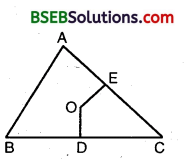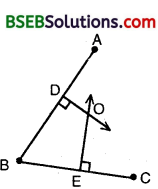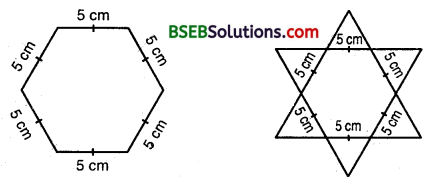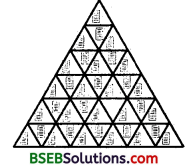# HSSlive: Plus One & Plus Two Notes & Solutions for Kerala State Board

## BSEB Class 9 Maths Chapter 7 Triangles Ex 7.5 Textbook Solutions PDF: Download Bihar Board STD 9th Maths Chapter 7 Triangles Ex 7.5 Book AnswersBSEB Class 9 Maths Chapter 7 Triangles Ex 7.5 Textbook Solutions PDF: Download Bihar Board STD 9th Maths Chapter 7 Triangles Ex 7.5 Book Answers

BSEB Class 9th Maths Chapter 7 Triangles Ex 7.5 Textbooks Solutions and answers for students are now available in pdf format. Bihar Board Class 9th Maths Chapter 7 Triangles Ex 7.5 Book answers and solutions are one of the most important study materials for any student. The Bihar Board Class 9th Maths Chapter 7 Triangles Ex 7.5 books are published by the Bihar Board Publishers. These Bihar Board Class 9th Maths Chapter 7 Triangles Ex 7.5 textbooks are prepared by a group of expert faculty members. Students can download these BSEB STD 9th Maths Chapter 7 Triangles Ex 7.5 book solutions pdf online from this page.

## Bihar Board Class 9th Maths Chapter 7 Triangles Ex 7.5 Books Solutions

 Board BSEB Materials Textbook Solutions/Guide Format DOC/PDF Class 9th Subject Maths Chapter 7 Triangles Ex 7.5 Chapters All Provider Hsslive

## How to download Bihar Board Class 9th Maths Chapter 7 Triangles Ex 7.5 Textbook Solutions Answers PDF Online?

2. Click on the Bihar Board Class 9th Maths Chapter 7 Triangles Ex 7.5 Answers.
3. Look for your Bihar Board STD 9th Maths Chapter 7 Triangles Ex 7.5 Textbooks PDF.
4. Now download or read the Bihar Board Class 9th Maths Chapter 7 Triangles Ex 7.5 Textbook Solutions for PDF Free.

Find below the list of all BSEB Class 9th Maths Chapter 7 Triangles Ex 7.5 Textbook Solutions for PDF’s for you to download and prepare for the upcoming exams:

## BSEB Bihar Board Class 9th Maths Solutions Chapter 7 Triangles Ex 7.5

Question 1.
ABC is A triangle. Locate a point in the interior of ∆ ABC which is equidistant from all the vertices of ∆ ABC.
Solution:
Let OD and OE be the A perpendicular bisectors of sides BC arid CA of ∆ ABC.∴ O is equidistant from two ends B and C of line-segment BC as O lies on the perpendicular bisector of BC.
Similarly, O is equidistant from C and A.
Thus, the point , of intersection O of the perpendicular bisectors of sides BC, CA and AB is the required point.

Question 2.
In a triangle locate a point in its interior which is equidistant from all the sides of the triangle.
Solution:
Let BE and CF be the bisectors of ∠ABC and ∠ACB respectively intersecting AC and AB at E and F respectively.Since. O lies on BE, the bisector of ∠ABC, hence O will be equidistant from AB and BC. Again, O lies on the bisector CF of ∠ACB. Hence, O will be equidistant from BC and AC. Thus, O will be equidistant from AB, BC and CA.

Question 3.
In a huge park, people are concentrated at three points (see figure):
A : where there are different slides and swings for children,
B : near which a man-made iake is situated,
C : which is near to a large parking and exit.
Where should an icecream parlour be set up so that maximum number of persons can approach it?
Solution:
The parlour should be equidistant from A, B and C, for which the point of intersection of perpendicular bisector should be located.Thus, O is the required point which is equidistant from A, B and C.

Question 4.
Complete the hexagonal and star Rangolies [see figure (i) and (ii)] by filling them with as many equilateral triangles of side 1 cm as you can. Count the number of triangles in each case. Which has more triangles ?Solution:
On filling each figure with equilateral triangles of side 1 cm, we find in figure (i) number of such triangles is 1∠50, and in figure (ii) number of such triangle is 300.## Bihar Board Class 9th Maths Chapter 7 Triangles Ex 7.5 Textbooks for Exam Preparations

Bihar Board Class 9th Maths Chapter 7 Triangles Ex 7.5 Textbook Solutions can be of great help in your Bihar Board Class 9th Maths Chapter 7 Triangles Ex 7.5 exam preparation. The BSEB STD 9th Maths Chapter 7 Triangles Ex 7.5 Textbooks study material, used with the English medium textbooks, can help you complete the entire Class 9th Maths Chapter 7 Triangles Ex 7.5 Books State Board syllabus with maximum efficiency.

## FAQs Regarding Bihar Board Class 9th Maths Chapter 7 Triangles Ex 7.5 Textbook Solutions

#### Can we get a Bihar Board Book PDF for all Classes?

Yes you can get Bihar Board Text Book PDF for all classes using the links provided in the above article.

## Important Terms

Bihar Board Class 9th Maths Chapter 7 Triangles Ex 7.5, BSEB Class 9th Maths Chapter 7 Triangles Ex 7.5 Textbooks, Bihar Board Class 9th Maths Chapter 7 Triangles Ex 7.5, Bihar Board Class 9th Maths Chapter 7 Triangles Ex 7.5 Textbook solutions, BSEB Class 9th Maths Chapter 7 Triangles Ex 7.5 Textbooks Solutions, Bihar Board STD 9th Maths Chapter 7 Triangles Ex 7.5, BSEB STD 9th Maths Chapter 7 Triangles Ex 7.5 Textbooks, Bihar Board STD 9th Maths Chapter 7 Triangles Ex 7.5, Bihar Board STD 9th Maths Chapter 7 Triangles Ex 7.5 Textbook solutions, BSEB STD 9th Maths Chapter 7 Triangles Ex 7.5 Textbooks Solutions,
Share: